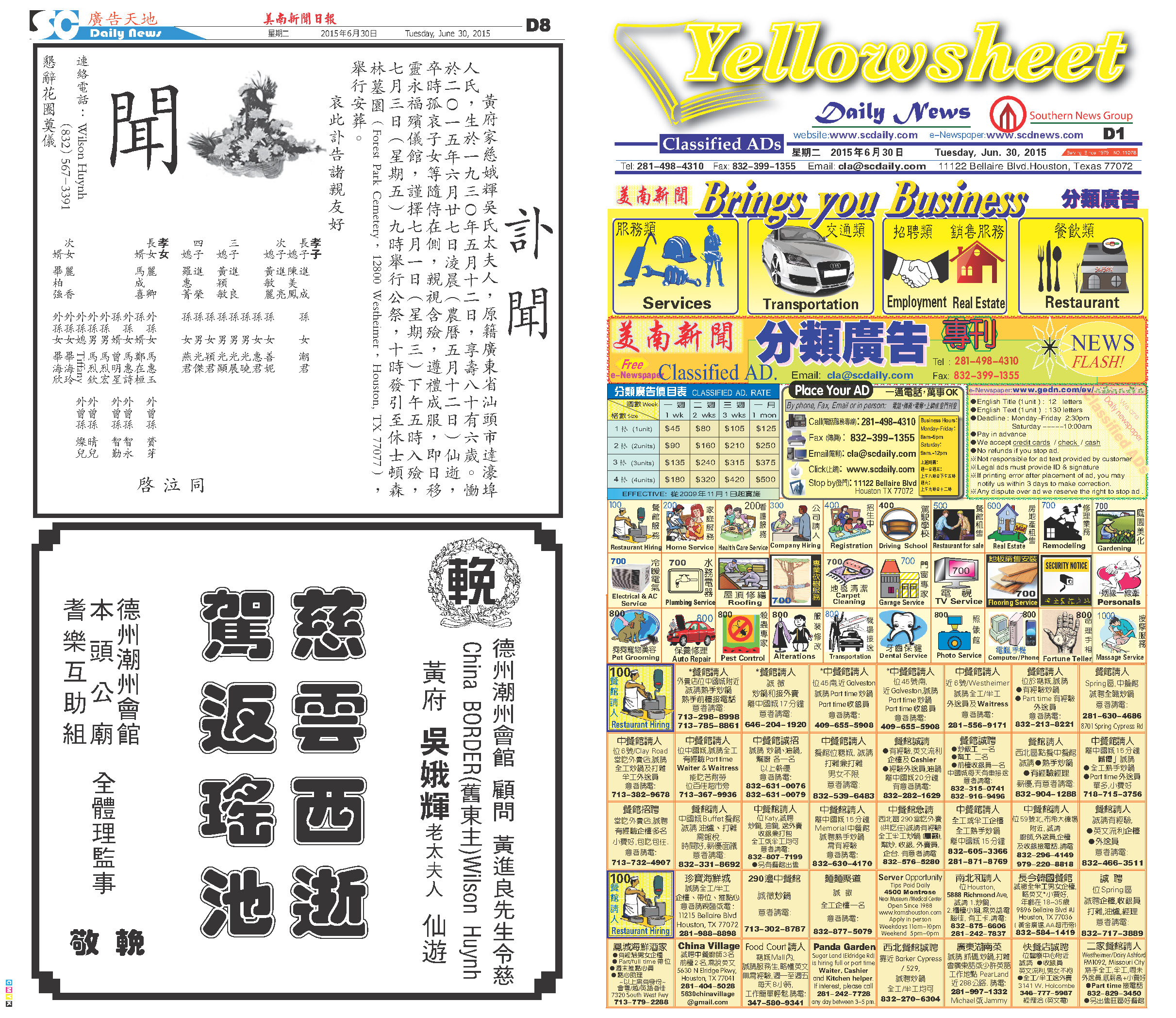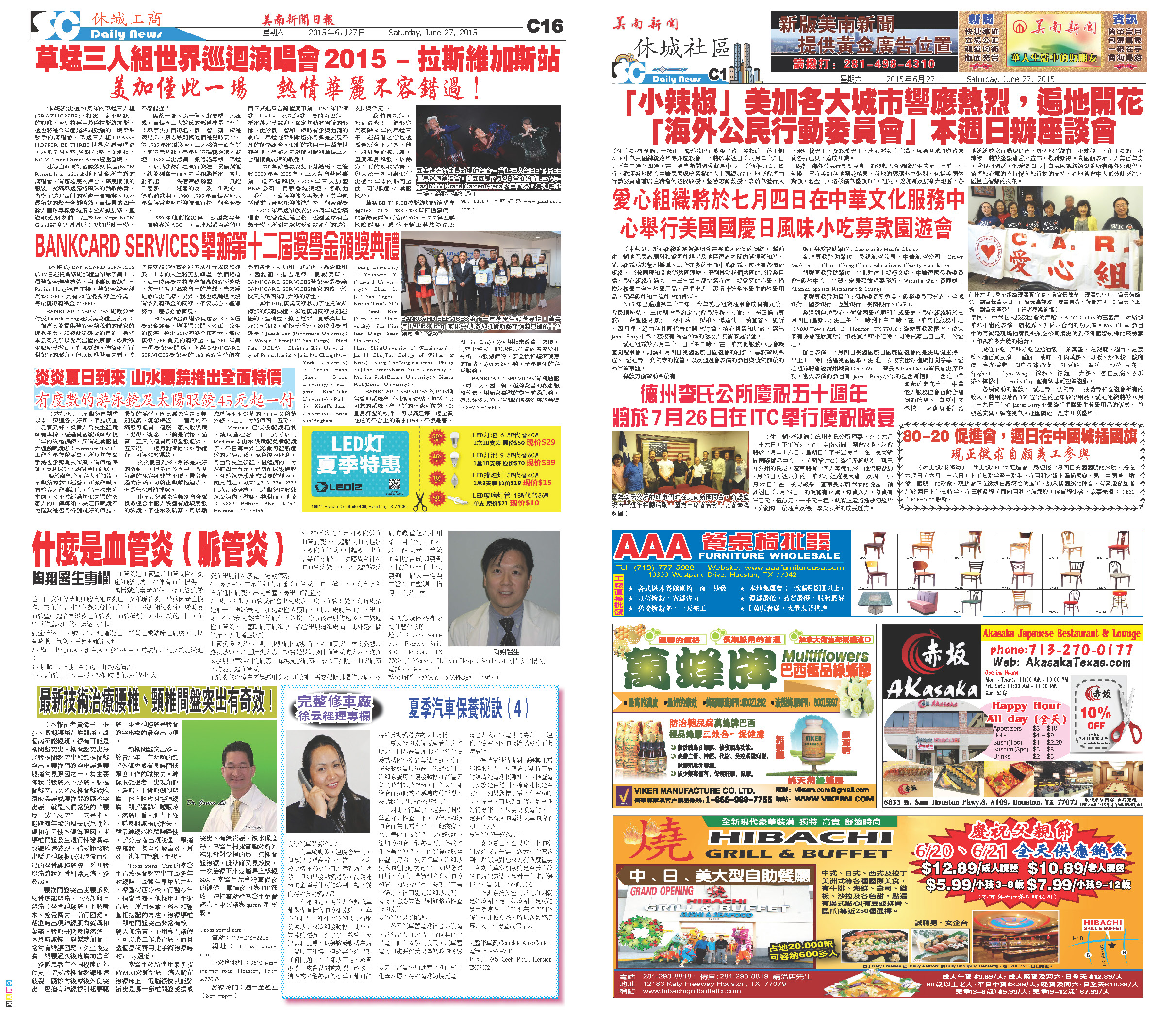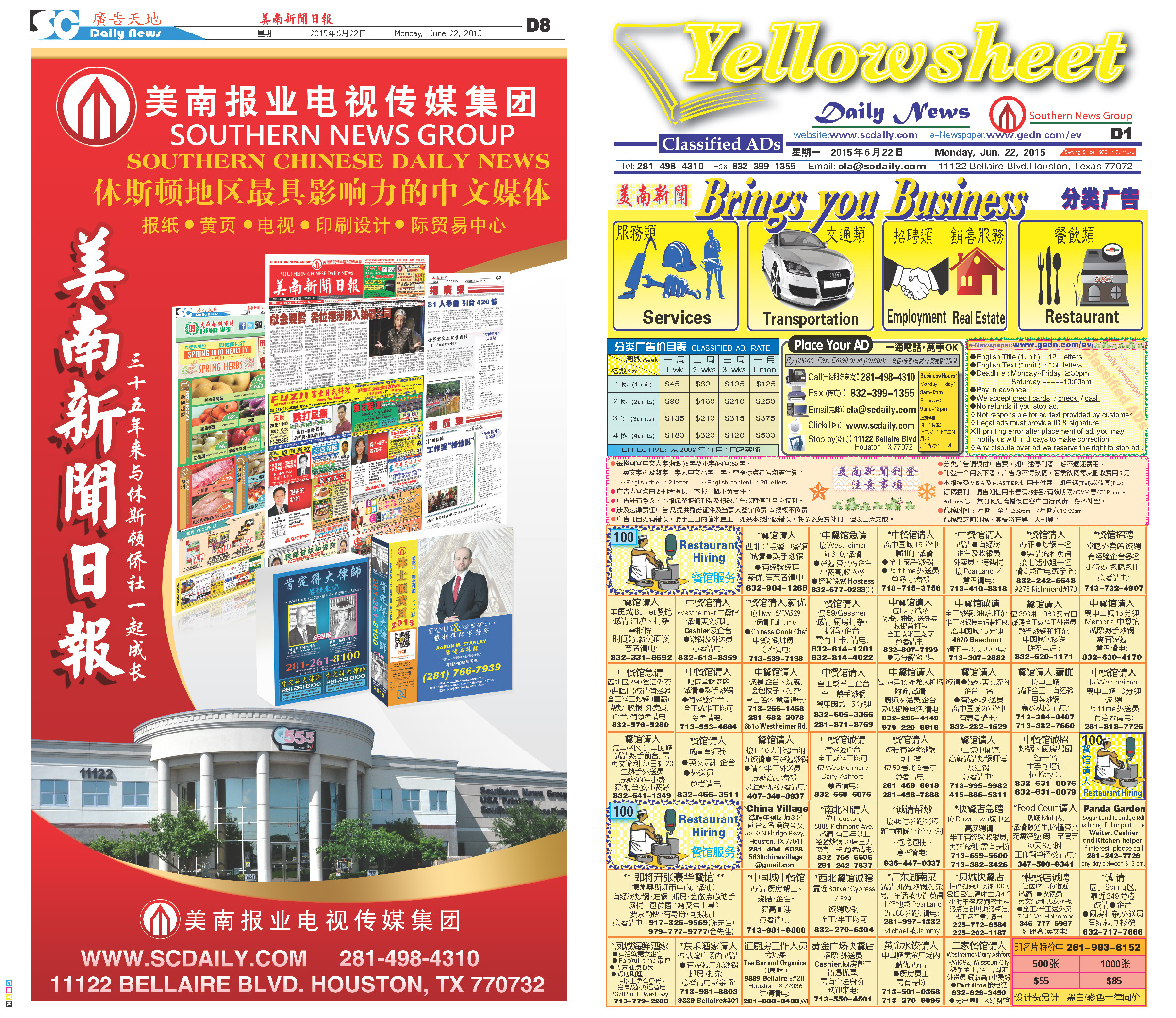150702 Epaper

 A Section B SectionC Section D Section150701 Epaper

 A Section B SectionC Section D Section150630 Epaper

 A Section B SectionC Section D Section150629 Epaper

 A Section B SectionC Section D Section150628 Epaper

 A Section B SectionC Section D Section150627 Epaper

 A Section B SectionC Section D Section150626 Epaper

 A Section B SectionC Section D Section150625 Epaper

 A Section B SectionC Section D Section150623 Epaper

 A Section B SectionC Section D Section150624 Epaper

 A Section B SectionC Section D Section150622 Epaper

 A Section B SectionC Section D Section